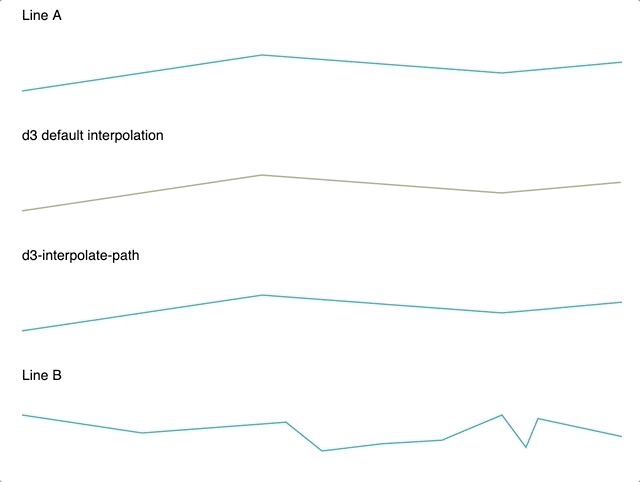# d3-interpolate-path

Interpolates path `d` attribute smoothly when A and B have different number of points.

## Usage no npm install needed!

``````<script type="module">
import d3InterpolatePath from 'https://cdn.skypack.dev/d3-interpolate-path';
</script>``````

# d3-interpolate-pathd3-interpolate-path is a zero-dependency that adds an interpolator optimized for SVG <path> elements. It can also work directly with object representations of path commands that can be later interpreted for use with canvas or WebGL.

Note this package no longer has a dependency on d3 or d3-interpolate, but in web usage adds itself to the d3 namespace like other d3 plugins do.## Example Usage

``````var line = d3.line()
.curve(d3.curveLinear)
.x(function (d) { return x(d.x); })
.y(function (d) { return y(d.y); });

d3.select('path.my-path')
.transition()
.duration(2000)
.attrTween('d', function (d) {
var previous = d3.select(this).attr('d');
var current = line(d);
return d3.interpolatePath(previous, current);
});
``````

If you're using it in a module environment, you can import it as follows:

``````import { interpolatePath } from 'd3-interpolate-path';
``````

Otherwise, you can use it via a `<script>` tag as follows:

``````<script src="https://unpkg.com/d3-interpolate-path/build/d3-interpolate-path.min.js"></script>
``````

## Development

Get rollup watching for changes and rebuilding

``````npm run watch
``````

Run a web server in the docs directory

``````cd docs
php -S localhost:8000
``````

Go to http://localhost:8000

## Installing

If you use NPM, `npm install d3-interpolate-path`. Otherwise, download the latest release.

## API Reference

# interpolatePath(a, b, excludeSegment)

Returns an interpolator between two path attribute `d` strings a and b. The interpolator extends a and b to have the same number of points before applying linear interpolation on the values. It uses De Castlejau's algorithm for handling bezier curves.

``````var pathInterpolator = interpolatePath('M0,0 L10,10', 'M10,10 L20,20 L30,30')
pathInterpolator(0)   // 'M0,0 L10,10 L10,10'
pathInterpolator(0.5) // 'M5,5 L15,15 L20,20'
pathInterpolator(1)   // 'M10,10 L20,20 L30,30'
``````

You can optionally provide a function excludeSegment that takes two adjacent path commands and returns true if that segment should be excluded when splitting the line. A command object has form `{ type, x, y }` (with possibly more attributes depending on type). An example object:

``````// equivalent to M0,150 in a path `d` string
{
type: 'M',
x: 0,
y: 150
}
``````

This is most useful when working with d3-area. Excluding the final segment (i.e. the vertical line at the end) from being split ensures a nice transition. If you know that highest `x` value in the path, you can exclude the final segment by passing an excludeSegment function similar to:

``````function excludeSegment(a, b) {
return a.x === b.x && a.x === 300; // here 300 is the max X
}
``````

# interpolatePathCommands(aCommands, bCommands, excludeSegment)

Returns an interpolator between two paths defined as arrays of command objects a and b. The interpolator extends a and b to have the same number of points if they differ. This can be useful if you want to work with paths in other formats besides SVG (e.g. canvas or WebGL).

Command objects take the following form:

``````| { type: 'M', x: number, y: number },
| { type: 'L', x, y }
| { type: 'H', x }
| { type: 'V', y }
| { type: 'C', x1, y1, x2, y2, x, y }
| { type: 'S', x2, y2, x, y }
| { type: 'Q', x1, y1, x, y }
| { type: 'T', x, y }
| { type: 'A', rx, ry, xAxisRotation, largeArcFlag, sweepFlag, x, y }
| { type: 'Z' }
``````

Example usage:

``````const a = [
{ type: 'M', x: 0, y: 0 },
{ type: 'L', x: 10, y: 10 },
];
const b = [
{ type: 'M', x: 10, y: 10 },
{ type: 'L', x: 20, y: 20 },
{ type: 'L', x: 200, y: 200 },
];

const interpolator = interpolatePathCommands(a, b);

> interpolator(0);
[
{ type: 'M', x: 0, y: 0 },
{ type: 'L', x: 5, y: 5 },
{ type: 'L', x: 10, y: 10 },
]

> interpolator(0.5);
[
{ type: 'M', x: 5, y: 5 },
{ type: 'L', x: 12.5, y: 12.5 },
{ type: 'L', x: 105, y: 105 },
]
``````

# pathCommandsFromString(pathDString)

Converts a path `d` string into an array of path command objects to work with interpolatePathCommands.

Example usage:

``````const a = 'M0,0L10,10';

> pathCommandsFromString(a)
[
{ type: 'M', x: 0, y: 0 },
{ type: 'L', x: 10, y: 10 },
]
``````